# Algebra

Welcome to Algebra! Below you will find a list of lessons, videos, and practice questions all relating to Algebra. If you’re looking to make math suck just a little bit less, think about Subscribing to our Youtube channel for the latest MathSux videos updated every week! Thanks for stopping by and happy calculating!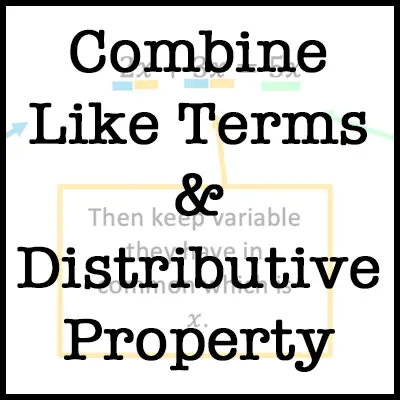Combining Like Terms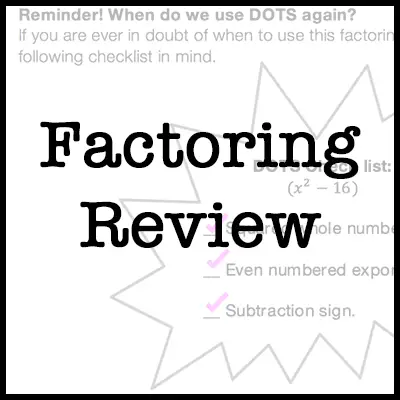Factoring Review4 Ways to Factor TrinomialsPiece-wise Functions (Regents)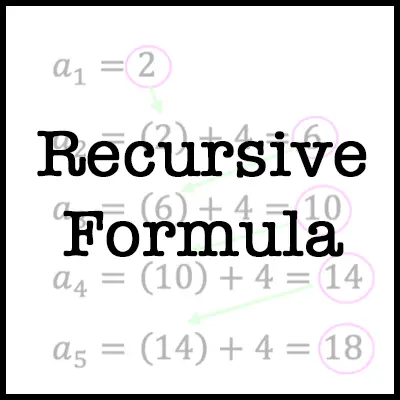Recursive Formulas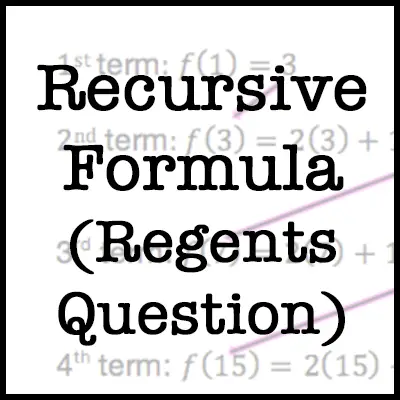Recursive Formula (Regents)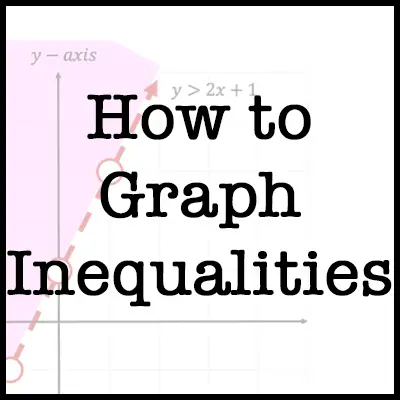How to Graph InequalitiesNYS Algebra Regents 2020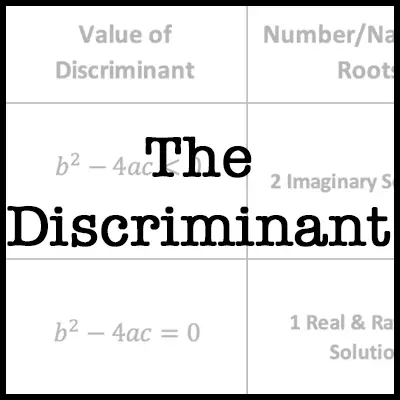The Discriminant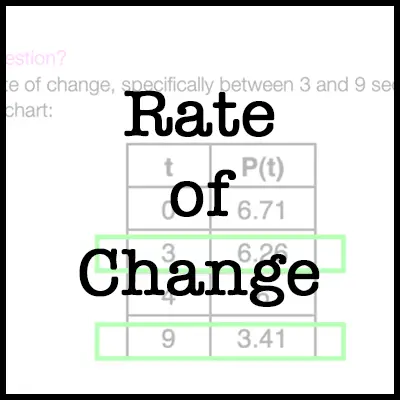Rate of Change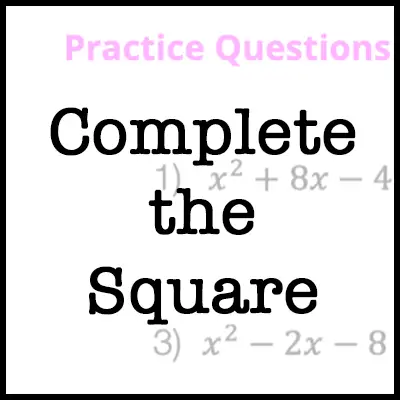How to Complete the Square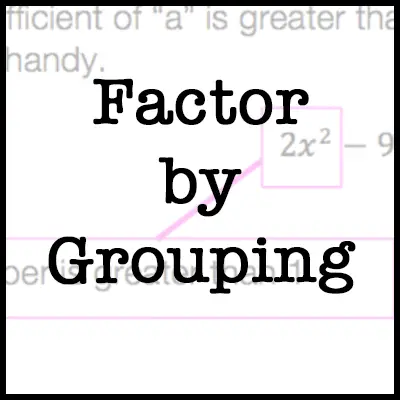Factor by Grouping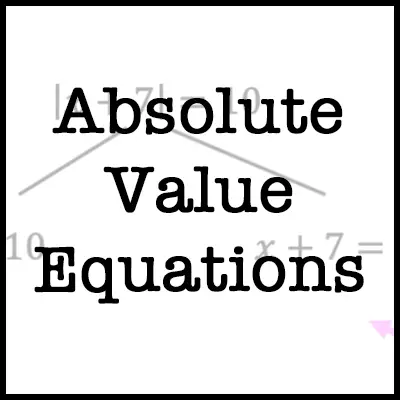Absolute Value Equations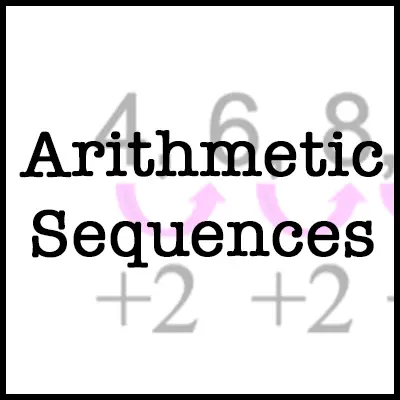Arithmetic Sequences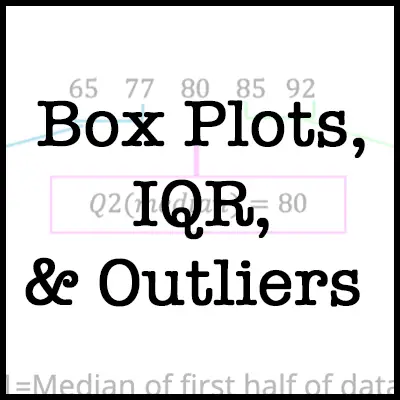Box Plots, IQR, & Outliers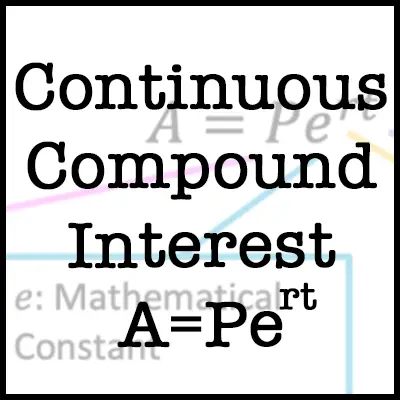Compound Interest Formula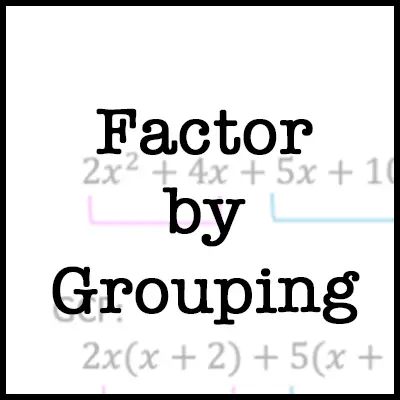Factor by Grouping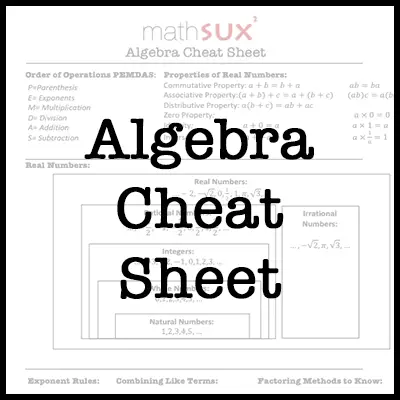Algebra Cheat Sheet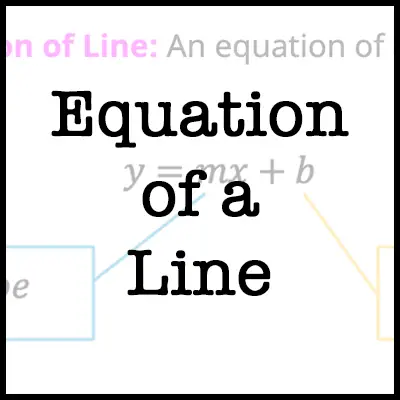How to Graph Equation of a Line y=mx+b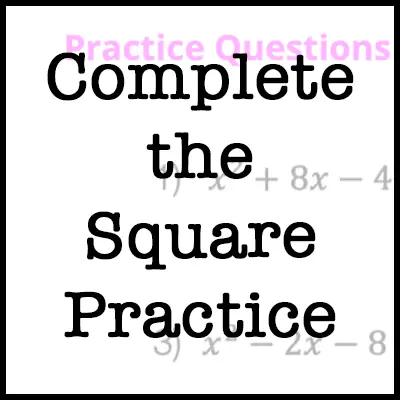Complete the Square practice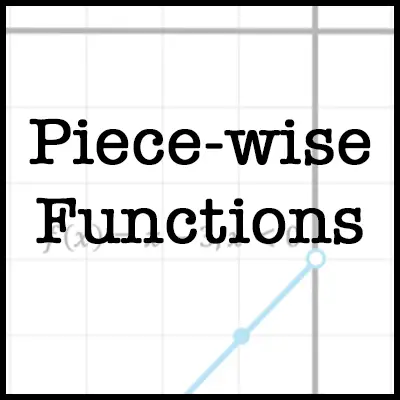Piecewise Functions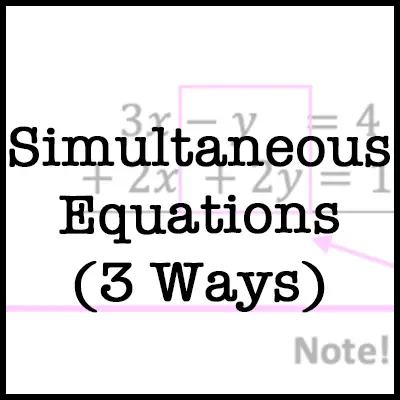Simultaneous Equations (3 Ways)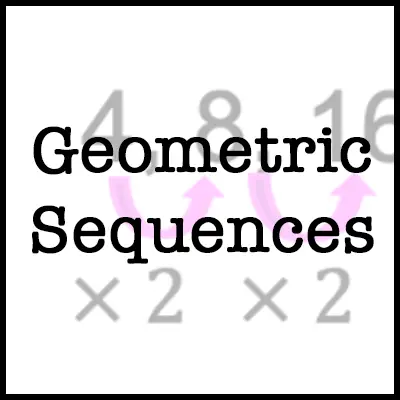Geometric Sequences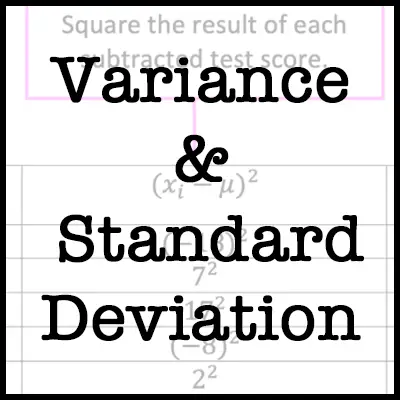Variance & Standard Deviation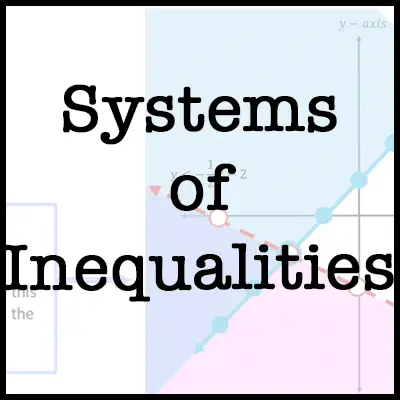Systems of Inequalities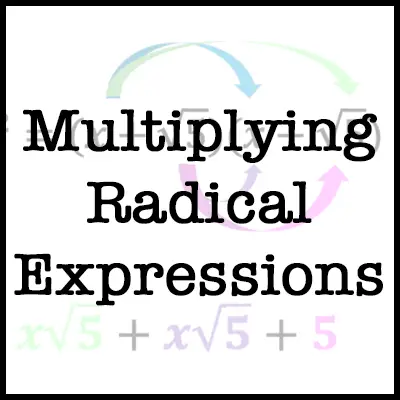Multiplying Radical Expressions

Looking for more lessons? Don’t forget to check out Algebra 2/Trig. here!